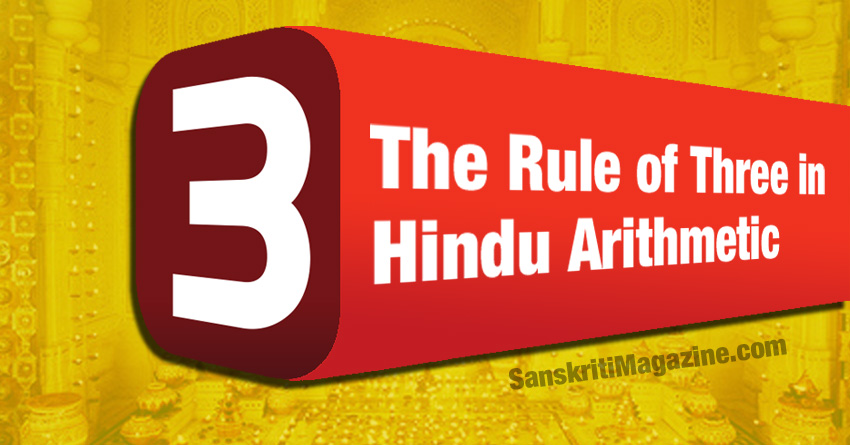Oops! It appears that you have disabled your Javascript. In order for you to see this page as it is meant to appear, we ask that you please re-enable your Javascript!
Vedic Science

# The Rule of Three in Hindu ArithmeticArithmetic is the science of numbers. The name comes from the Greek word ‘arithmos’ that means ‘number’. In India, arithmetic was called Raasi-Vidyaa, Samkyaana orGananaa and was known from very ancient times.

Here and now, I will say a few things about the Rule of Three. The Hindu name for the Rule of Three was trairaasikaa and the method is an ancient one having been known in Greater India (Bhaarata) to Vedic mathematicians. It occurs first in Bakshaali Manuscript near about the beginning of Christian era and subsequently in the writings of Aryabhatta I (499 CE), Brahmagupta (628 CE), Sridhara (c.750), Mahavira (850 CE), Aryabhatta II (950 CE), Bhaskara II (1150 CE).

## THE RULE OF THREE:

Rule of Three is a fast method for mechanically solving arithmetic problems in proportion without the knowledge of the general theory of proportion. What the rule says is this: knowing three quantities A, B, C, we can determine the fourth unknown quantity directly without resorting to proportion or the use of the variable ‘x’, that is without taking recourse to algebra. Write the quantities A, B, C in a row, a line or linear sequence.

Now read the quantities in reverse order multiplying the last term C by the middle term B and dividing the product BC by the first term A to straightaway arrive at the answer. While writing the numbers in the linear sequence, make sure that A and C are of one kind (units) and B (second quantity, number or term) is of the same kind as the unknown quantity or number.

The Hindu number theorists gave the name Pramaana to A, Phala to B and C was named Icchaa.

Problem 1: If 4 metres of cloth cost 100 rupees, how much will 10 metres of cloth cost? Here A=4, B=100 and C=10. We immediately write the answer as 100×10/4 = 250 rupees.

Problem 2: If 4 metres of cloth cost 100 rupees, how much cloth can be bought in 250 rupees? Here the three numbers are: A=100, B=4 and C= 250. The answer to the question is BC/A = 4×250/100 = 10 metres.

## CONCLUSIONS:

The rule was perfected among Indian (Hindu) mathematicians during the early centuries of the Christian era. It then travelled to the Arabs in the 8th century C.E. and from them to Europe where it was hailed as the “Golden Rule” during Renaissance times. Both the Arabs and the Europeans adopted the Hindu name ‘The Rule of Three’.

They also adopted the practice of placing the terms in a line and kept the first and the last similar. But the names of the three terms were altered. This is understandable because the names were in Sanskrit and had they retained the same names, the source would have been known. So the method was taken but the terms were renamed.

The importance of this Rule lies in the simplicity of the process which results in reduction of the number of mathematical steps or operations. Whenever and wherever, repeated calculations in proportions are required such as in commercial problems, the method would save a lot of unnecessary thinking and time. The method can be applied on a slide rule (Ref: The Mechanic’s Calculator by William Grier).

Since, The Rule of Three is a shorthand version of proportionality calculations, it can not be ruled out that the ancient Sanskrit writers did not know the theory behind the method. The Rule of Three occupies an interesting place in the history of transmission of mathematical ideas.

BY DR. SACHIDANAND  DAS , PhD (Science, 1981, Bombay), BSc (Gold Medalist,   Loyola College, Chennai, 1964), BSc (English, Sanskrit, Mathematics, 1964), SERC Senior Visiting Fellow, London (1984-86), CSci (Institute of Physics, London, 1985), Distinguished Leadership Award and Advisor (RBI, USA), (Nom.) Member of New York Academy of Sciences (1995) and Man of the Year by RBI(1997), Certificate From World Pranic Healing Foundation, Inc (1999), Franklin Templeton Man of The Match Award (2015).

#### 1 Comment

This site uses Akismet to reduce spam. Learn how your comment data is processed.

•Sagar says:

Salute…

#### Get daily updates via Email

Enter your email address:

## Sanskrit Mantras- How it works? A Scientific Perspective